# Case Study: Transfer Learning for Gender Detection

Alex Egg,

In this post we will use Tensorflow to fine-tune a Caffe face detection model for the task of Gender detection.

Code and Jupiter Notebook on GitHub: https://github.com/eggie5/Transfer-Learning-For-Gender-Detection

## Introduction

We want to make a gender detector where the model input is an image of a face and the output is the Gender: Male, Female or Other.

Our first step is the data prep: We need facial images w/ labeled gender. Instead of curating a crowd-sourced facial gender image annotation study/task, we can leverage an academic dataset: Facial Image Dataset 1 The dataset has 26k labeled images of 2.2k subjects. We could probably train a classifier from scratch w/ this amount of images, but training from scratch has two drawbacks:

• You will risk overfitting if there is not enough data. In other words, there might not be enough images (data) to generalize well enough. For example, a typical image-net classifier is trained on 1M images!
• We might not have enough compute power to train a Deep CNN arch on 26k images. Do you have a GPU?

These two drawbacks motivate a good case for Transfer Learning.

## Transfer Learning Theory

We know that sequential layers in a CNN arch build progressively more semantic representations. For example, the early layer may learn edges or shapes while the latter layers may learn higher level objects.

An example of this can be seen below in figure 1. Figure c & d, from the DeCAF paper shows the clustering of ImageNet categories in 2 dimensions, where (c) is features from the first pooling layer and d is from the penultimate layer. Clearly defined clusters in d, shows how the first layers learn low-level features, whereas the latter layers learn semantic or more high-level features.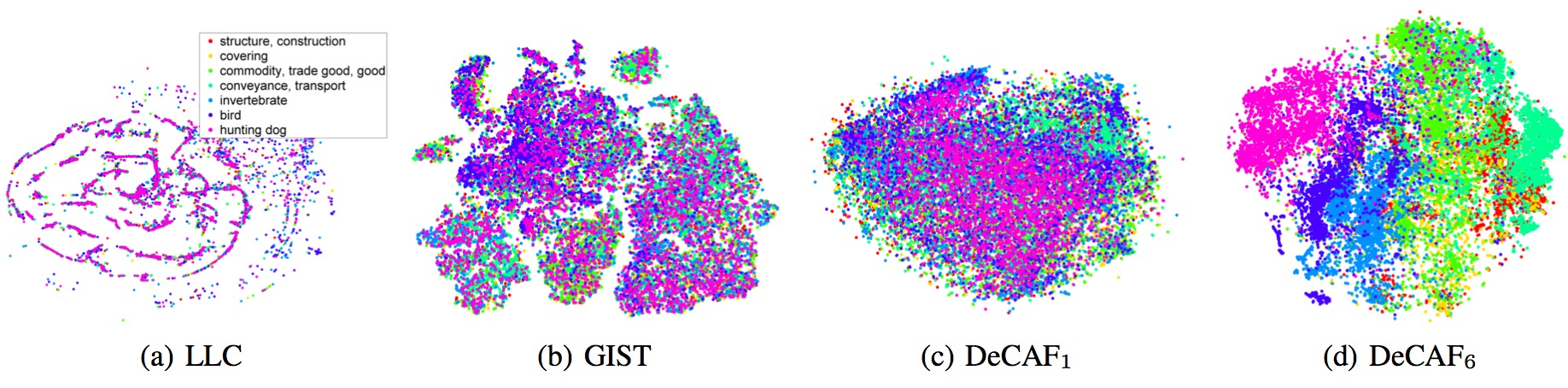Figure 1: Figure c & d, from the DeCAF paper shows the clustering of ImageNet categories in 2 dimensions, where c is features from the first pooling layer and d is from the penultimate layer.

Another example, in Figure 2, you can see the semantic representation possible w/ a CNN. This VGG16 CNN was trained on dataset of Amazon Women’s Clothing images. The interesting thing to note is that there was no clustering algorithm run on this data, it is the pure output of the Convolutional Layers which would suggest that the network has learned some semantic representation of what are various clothing items.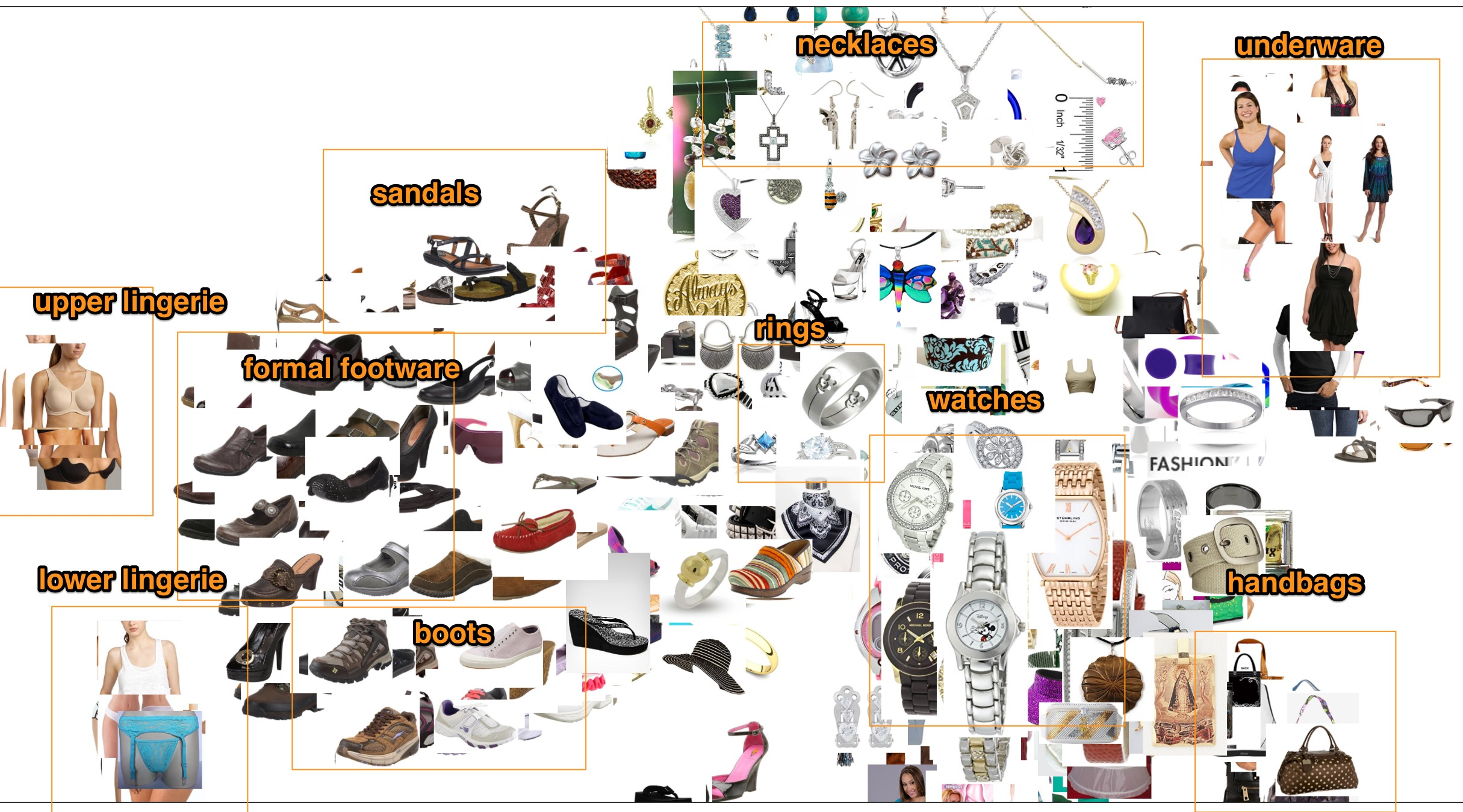Figure 2: Embeddings from final Conv. Layer of a VGG16 Network projected in to 2D.

So with this technical understand of CNNs we can gain the intuition that if we had a previously trained model that is very good at one task, maybe we can adapt it to another task. For example if we can find model that is very good at facial detection, maybe we can just tweak it a bit to do gender detection.

This also aligns with the philosophy of supervised transfer: one may view the trained model as an analog to the prior knowledge a human obtains from previous visual experiences, which helps in learning new tasks more efficiently. 2

There are two ways to do the general technique of transfer learning:

1. Use the CNN outputs (embeddings) as input features for a separate (gender) classifier.
2. Fine Tune the pertained model for the new task

### Feature Extractor

This is the easiest method with will use our pertrained model as a feature extractor for our new classifier. You just run all your images through the CNN and save the output embeddings and then use those for a inputs to your new model on the novel task.

### Fine Tuning

This technique modifies the pretrained network for your novel task. For example, a pretrained imagenet model will have a 1000D softmax on the end. If your new task is hotdog/not hotdog you only need 1 neuron on the end and not 1000, so you can modify the network to fit that need and retrain it for a few epochs on the new hotdog images. This Joint Training technique has the benefit of optimizing the whole or at least a portion of the pre-trained model to match the distribution of the new data.

This is where there are a number of techniques for retraining.

• Retrain only the softmax layer
• Retrain any number of the FC layers
• Retrain all the layers (w/ a slow learning rate)

Or you can do a combination of these techniques, for example, first retrain the FCs for a few epochs, then retrain the whole network for a few epochs.

Regardless here is the general technique:

2. Freeze the base network.
3. Train the part you added.
4. Unfreeze some layers in the base network.
5. Jointly train both these layers and the part you added.

## Gender Detector (Fine Tuning)

Since we are afraid of training a model from scratch due to the lack of image data from our gender set we will use transfer learning. We can leverage an academic model based on the famous VGG16 CNN architecture called VGG Face3 . Compared to our gender dataset which only had 26k images and 2.2k subjects, the VGG Face model was trained on 2.6M images of 2.6k subjects!Figure 3: VGG Architecture with 12 Convolutional Layers and 3 FC Layers and Softmax at the end. 4

The VGG Face project release the VGG weights in Caffe, Matlab and torch formats. I’ve seen some projects in the community that can convert Caffe to Tensorflow.

### Caffe to TF Conversion

I know a popular solution in the community is the caffe-tensorflow project. In order to do the conversion you need to have a working Caffe environment w/ python bindings. I used the docker image blvc/caffe :

docker run -ti -v ~/Development/workspace/matroid/vgg_face_caffe/:/root/shared_folder bvlc/caffe:cpu bash


First, we need to update the legacy Caffe syntax that comes with the VGG Face distribution:

upgrade_net_proto_text VGG_FACE_deploy.prototxt VGG_FACE_deploy.prototxt2


Run conversion:

caffe-tensorflow/convert.py VGG_FACE_deploy.prototxt2 --code-output-path VGG_FACE_deploy.py --caffemodel VGG_FACE.caffemodel --data-output-path VGG_FACE.npy

Type                 Name                                          Param               Output
----------------------------------------------------------------------------------------------
Input                input                                            --     (1, 3, 224, 224)
Convolution          conv1_1                                          --    (1, 64, 224, 224)
Convolution          conv1_2                                          --    (1, 64, 224, 224)
Pooling              pool1                                            --    (1, 64, 112, 112)
Convolution          conv2_1                                          --   (1, 128, 112, 112)
Convolution          conv2_2                                          --   (1, 128, 112, 112)
Pooling              pool2                                            --     (1, 128, 56, 56)
Convolution          conv3_1                                          --     (1, 256, 56, 56)
Convolution          conv3_2                                          --     (1, 256, 56, 56)
Convolution          conv3_3                                          --     (1, 256, 56, 56)
Pooling              pool3                                            --     (1, 256, 28, 28)
Convolution          conv4_1                                          --     (1, 512, 28, 28)
Convolution          conv4_2                                          --     (1, 512, 28, 28)
Convolution          conv4_3                                          --     (1, 512, 28, 28)
Pooling              pool4                                            --     (1, 512, 14, 14)
Convolution          conv5_1                                          --     (1, 512, 14, 14)
Convolution          conv5_2                                          --     (1, 512, 14, 14)
Convolution          conv5_3                                          --     (1, 512, 14, 14)
Pooling              pool5                                            --       (1, 512, 7, 7)
InnerProduct         fc6                                              --      (1, 4096, 1, 1)
InnerProduct         fc7                                              --      (1, 4096, 1, 1)
InnerProduct         fc8                                              --      (1, 2622, 1, 1)
Softmax              prob                                             --      (1, 2622, 1, 1)
Converting data...
Saving source...
Done.


The output of this conversion is an graph file and weights:VGG_FACE_deploy.py and VGG_weights.npy

from kaffe.tensorflow import Network
class VGG_FACE_16_layers(Network):
def setup(self):
(self.feed('input')
.conv(3, 3, 64, 1, 1, name='conv1_1')
.conv(3, 3, 64, 1, 1, name='conv1_2')
.max_pool(2, 2, 2, 2, name='pool1')
.conv(3, 3, 128, 1, 1, name='conv2_1')
.conv(3, 3, 128, 1, 1, name='conv2_2')
.max_pool(2, 2, 2, 2, name='pool2')
.conv(3, 3, 256, 1, 1, name='conv3_1')
.conv(3, 3, 256, 1, 1, name='conv3_2')
.conv(3, 3, 256, 1, 1, name='conv3_3')
.max_pool(2, 2, 2, 2, name='pool3')
.conv(3, 3, 512, 1, 1, name='conv4_1')
.conv(3, 3, 512, 1, 1, name='conv4_2')
.conv(3, 3, 512, 1, 1, name='conv4_3')
.max_pool(2, 2, 2, 2, name='pool4')
.conv(3, 3, 512, 1, 1, name='conv5_1')
.conv(3, 3, 512, 1, 1, name='conv5_2')
.conv(3, 3, 512, 1, 1, name='conv5_3')
.max_pool(2, 2, 2, 2, name='pool5')
.fc(4096, name='fc6')
.fc(4096, name='fc7')
.fc(2622, relu=False, name='fc8')
.softmax(name='prob'))


This is the VGG architecture w/ a 2622D softmax layer for the original face detection task.

We can load the pre-trained model like this:

net = VGG_FACE_16_layers({'input': images})


Where input is the graphs input placeholder.

### Modify the Network

We need to modify the softmax layer of the VGG Face arch for our new Gender task. This means we need to do 2 things:

1. Change 2622 neurons to 3 neuros in the Softmax
2. Restore the pre-trained weights to the base layers
print("Building graph...")
net = VGG_FACE_16_layers({'input': images}, num_classes=3)
logits = net.layers["fc8"]

fc8_variables = tf.contrib.framework.get_variables('fc8')
fc8_init = tf.variables_initializer(fc8_variables)

loss = tf.reduce_mean(tf.nn.sparse_softmax_cross_entropy_with_logits(logits=logits, labels=labels))
fc8_train_op = opt.minimize(loss, var_list=fc8_variables) #we only want to update FC8 ala fine-tuning

saver = tf.train.Saver()
print("...done")


The above code sets the softmax Layer to have 3 neuron and tells the TF optimizer to only update the last fully connected layer and the softmax. Later in the training loop we will restore only the weights put FC8:

with tf.Session() as sess:
sess.run(tf.global_variables_initializer())
sess.run(fc8_init)  # initialize the new fc8 layer
net.load(args.model_path, sess, scratch_layers=["prob", "fc8"]) #restore weights except for last FC
print("...done")

for epoch in range(args.num_epochs):
print('\nStarting epoch %d / %d' % (epoch + 1, args.num_epochs))
sess.run(train_init_op)

epoch_losses=[]
while True:
try:
_, xent = sess.run([fc8_train_op, loss])
epoch_losses.append(xent)
except tf.errors.OutOfRangeError:
break

#save model
print("Saved model to ckpts dir")
save_path = saver.save(sess, "./ckpts/model.ckpt")


### Training

I only trained the model on 1/5 of the training data or approx. 4.4k images and gender labels.

The Learning Curves are below in Figure 4. I also included the score before the network starts training or when the softmax weights are random.

The interesting thing to note is that after 1 training epoch the train and test accuracy pretty much converges! That’s the power of transfer learning: strong initiation.

Rand 1 2 3 4 5 6 7 8 9 10
Train 0.243310 0.876004 0.914139 0.956289 0.968555 0.978368 0.978591 0.978145 0.978814 0.980821 0.985727
Test 0.309115 0.794370 0.805630 0.830563 0.837265 0.842627 0.833512 0.830563 0.825201 0.832976 0.830831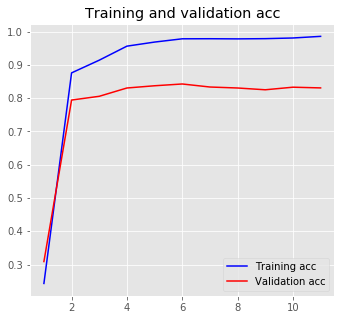Figure 4: Train and test learning curves over 10 epochs. The first data point is random weights before the first training epoch.

You can see an example of this phenomena in the Decaf paper too:

Also, very interesting results showing in Figure 5, show that the transfer learning model can get meaningful inferences with only 1 examples per class when non-transfer learned-standard ML models can’t get meaningful results until at least 10 examples per class:

They also highlight the point again that the new training data can be very sparse if the base model generalises well. For example in some cases categories w/ only 1 training example still achieve desirable results

“Our one-shot learning results (e.g., 33.0% for SVM) suggest that with sufficiently strong representations like DeCAF, useful models of visual categories can often be learned from just a single positive example.”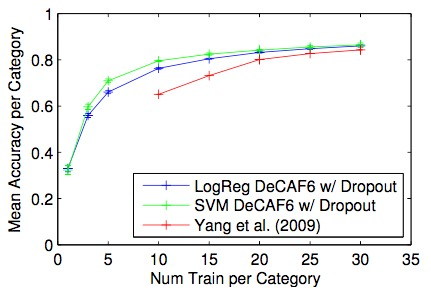Figure 5: Figure 5: Necessary examples per class to get inference results. See Transfer Learning models can start w/ only 1 example per class.

### Evaluation

We can look at standard multi-class evaluation techniques like top-k accuracy. However, in this case there are only 3 classes so we’ll just look at overall accuracy. However, depending our our requirements, we might be interested in other characteristics such as false-postive rates. For example, is it more expensive to misclassify a woman as a man or a man as a women? We can get insights into this by looking at the confusion matrix: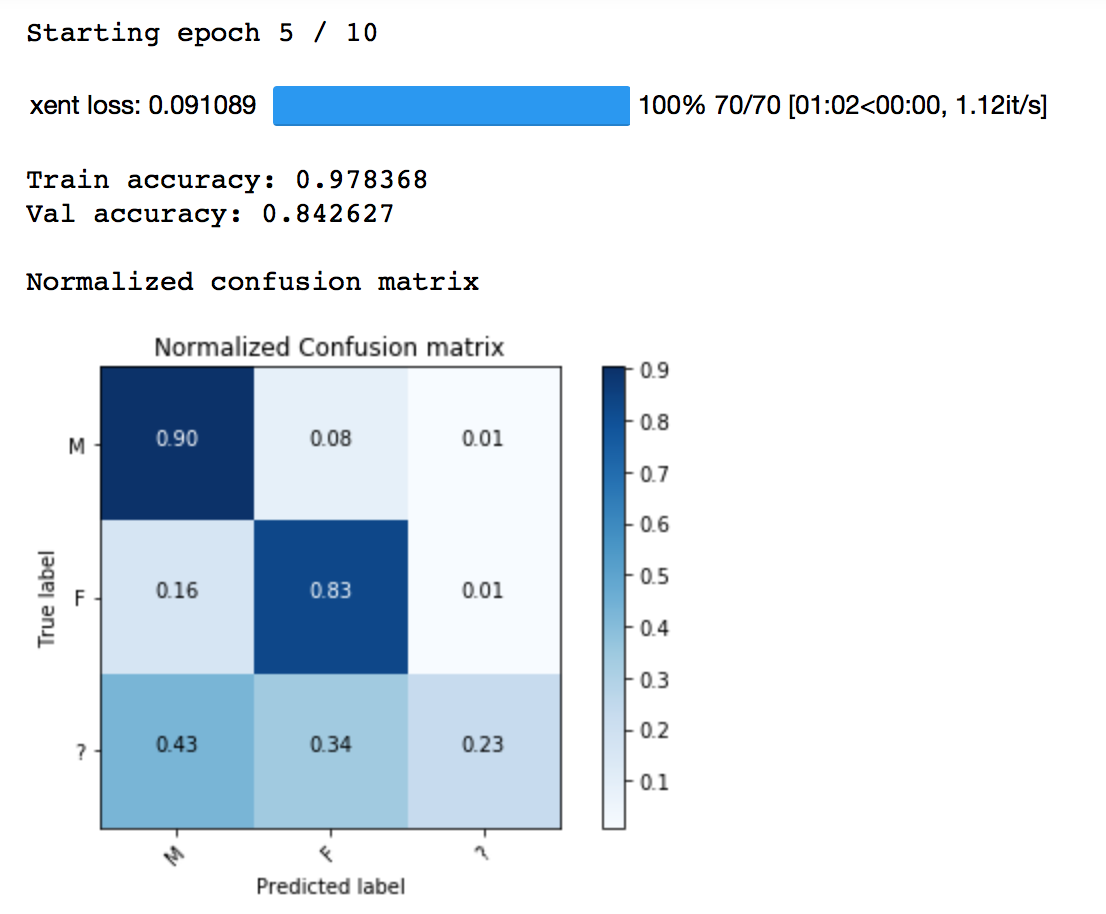For example, on the 5th epoch, you can see the overall accuracy was 84% but is that good or not? We can see that 90% of the time we can correctly classify a Male and that 85% of the time we can correctly classify a Female. However, 16% of the time we confuse a Female as a Male, but not as frequently the other way around which I think is intuitive b/c men have long hair much more frequently than women having short. Maybe your business objective is dependent on not minimizing Female confusion.

## Conclusion

We were able to take a model trained on Facial Recognition and modify it for the Novel task of Gender Detection. Since we used transfer learning and the new domain wasn’t very different from the original domain, we only had to train on a small sample of the gender data. We achieved 85% overall accuracy in about 5 minutes of training due to strong initiation which is a product of transfer learning.